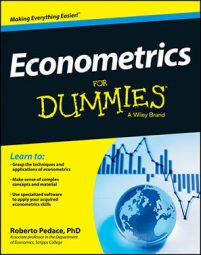##### Econometrics For DummiesEconometric techniques are used to estimate economic models, which ultimately allow you to explain how various factors affect some outcome of interest or to forecast future events. The ordinary least squares (OLS) technique is the most popular method of performing regression analysis and estimating econometric models, because in standard situations (meaning the model satisfies a series of statistical assumptions) it produces optimal (the best possible) results.

The proof that OLS generates the best results is known as the Gauss-Markov theorem, but the proof requires several assumptions. These assumptions, known as the classical linear regression model (CLRM) assumptions, are the following:

• The model parameters are linear, meaning the regression coefficients don't enter the function being estimated as exponents (although the variables can have exponents).

• The values for the independent variables are derived from a random sample of the population, and they contain variability.

• The explanatory variables don't have perfect collinearity (that is, no independent variable can be expressed as a linear function of any other independent variables).

• The error term has zero conditional mean, meaning that the average error is zero at any specific value of the independent variable(s).

• The model has no heteroskedasticity (meaning the variance of the error is the same regardless of the independent variable's value).

• The model has no autocorrelation (the error term doesn't exhibit a systematic relationship over time).

If one (or more) of the CLRM assumptions isn't met (which econometricians call failing), then OLS may not be the best estimation technique. Fortunately, econometric tools allow you to modify the OLS technique or use a completely different estimation method if the CLRM assumptions don't hold.